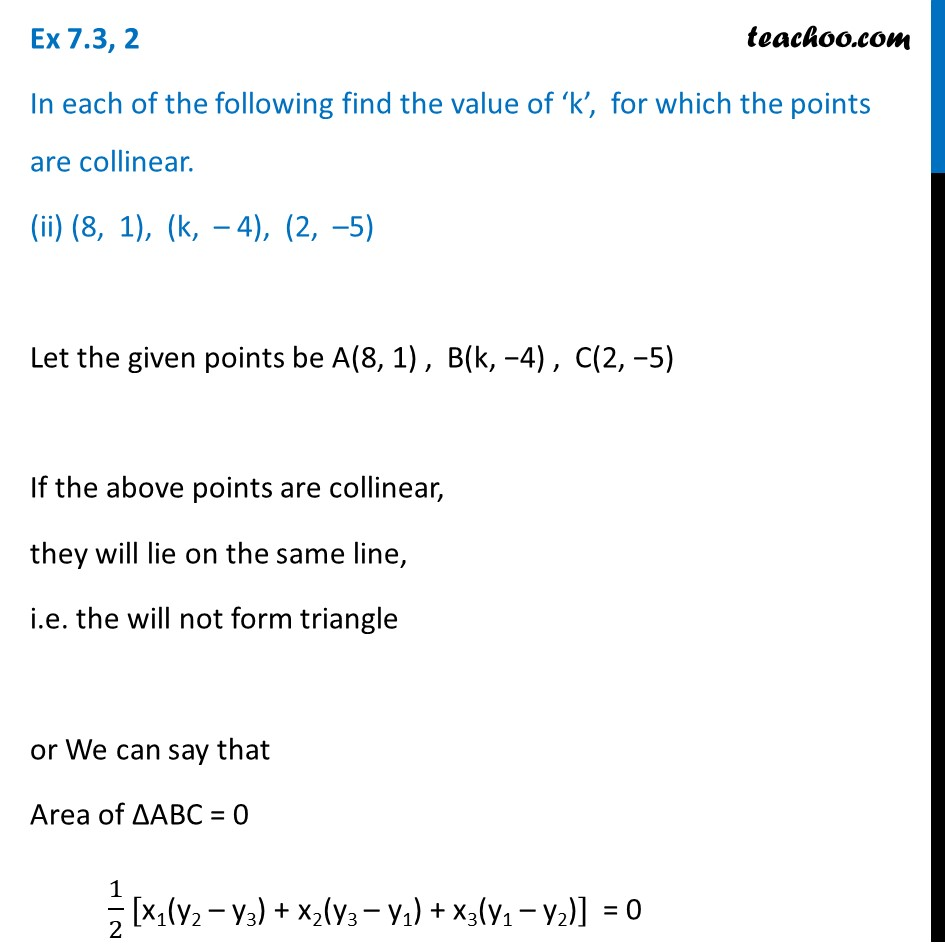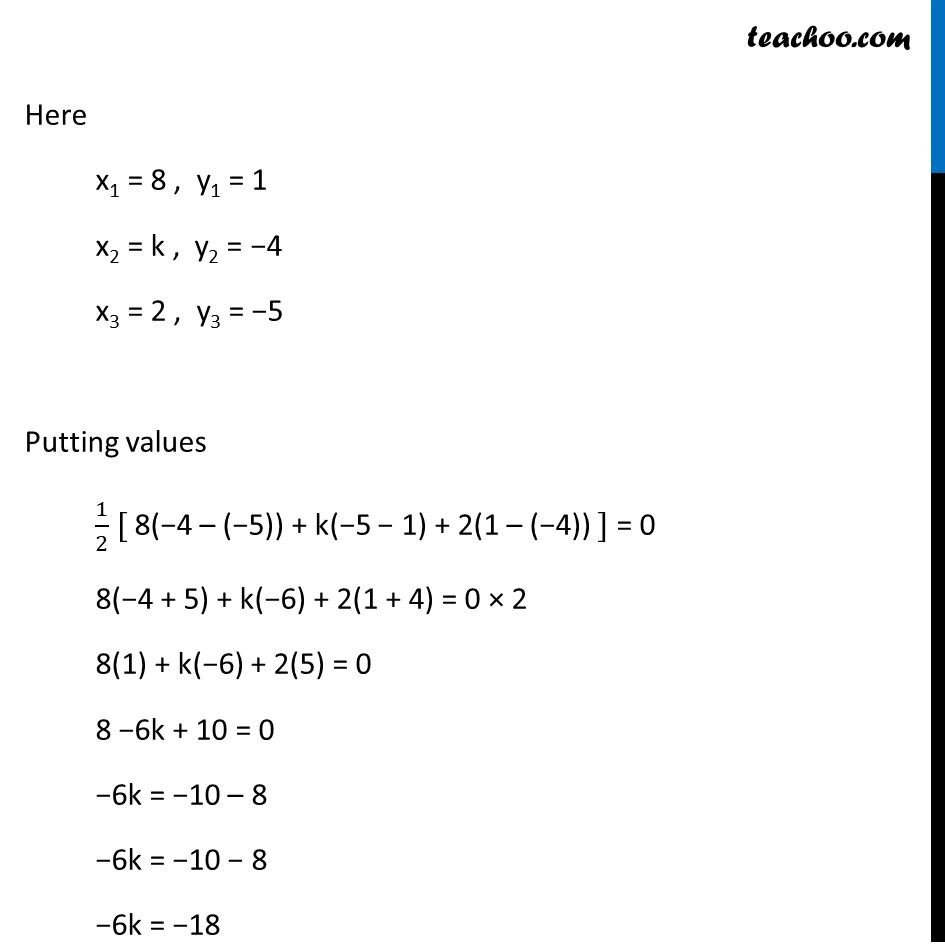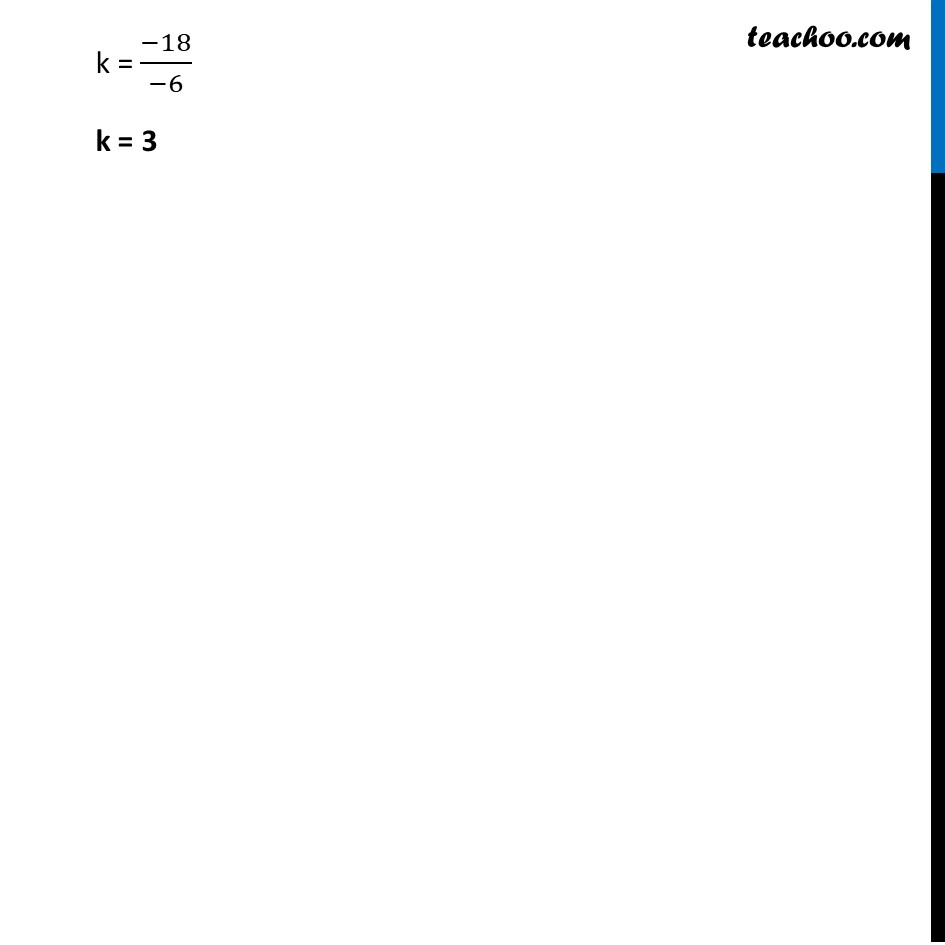Ex 7.3

Chapter 7 Class 10 Coordinate Geometry
Serial order wise### Transcript

Ex 7.3, 2 In each of the following find the value of ‘k’, for which the points are collinear. (ii) (8, 1), (k, – 4), (2, –5) Let the given points be A(8, 1) , B(k, −4) , C(2, −5) If the above points are collinear, they will lie on the same line, i.e. the will not form triangle or We can say that Area of ∆ABC = 0 1/2 [x1(y2 – y3) + x2(y3 – y1) + x3(y1 – y2)] = 0 Here x1 = 8 , y1 = 1 x2 = k , y2 = −4 x3 = 2 , y3 = −5 Putting values 1/2 [ 8(−4 – (−5)) + k(−5 − 1) + 2(1 – (−4)) ] = 0 8(−4 + 5) + k(−6) + 2(1 + 4) = 0 × 2 8(1) + k(−6) + 2(5) = 0 8 −6k + 10 = 0 −6k = −10 – 8 −6k = −10 − 8 −6k = −18 k = (−18)/(−6) k = 3